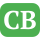CppBuzz.com

# Arrays in C programming - Single, Double & Multidimensional

Group of similar type of elements is known as array in programming world. Array can be of predefined or use defined data types.

## Points to remember about Array

• Array length can be any positive int value
• We can also create array of pointer
• We can access any element by its index value
• Array size can't in increased at runtime
• Size of Array is sum of size of its all elements
• We can create Array of any data type
• Types of Array - Single, Double & Multidimensional

## Declaration of integer Array

```int arr;
```

Here "arr" is the array which can contain 71 elements of int type

## Initialize Array

• Direct Initialization
• Initialization using user input
• Initialization using loops

## Direct Initialization of int Array

```int arr;

int a = 1;
int a = 2;
int a = 3;
int a = 5;
-------------
-------------
arr = 72;
```

a is known as first element of array, arr is known as second element of array. The last index of array would be n-1 i.e 70 here.

## Initialize int Array using loop

Array can be initialized using any of three loop ( for, while, do while ).

```#include <stdio.h>

int main()
{
int arr;
int i;

for(i=0; i<71; i++){
arr[i] = 1;
}

/* printing all of its elements */

for(i=0; i<71; i++){
printf(" %d ", arr[i]);
}

return 0;
}
```

## Initialize int Array using user input

```#include <stdio.h>

int main()
{
int arr;
int i;

for(i=0; i<2; i++){
scanf("%d",&arr[i]);
}

/* printing all of its elements */

for(i=0; i<2; i++){
printf(" %d ", arr[i]);
}

return 0;
}
```

## Size of Array

Size of any data type ( predefined or user defined ) can be calculated using the C library operator "sizeof()". Please note it is not a function, it is operator.
If we want to calculate without this operator then we can do manually like if array size is 71 like in above example arr then the size required is 71 * Size of each element. So if the size of int is 4 bytes then the size of "arr" would be 71 * 4 = 284 bytes. Hence the formula is Size of Array * Bytes required for each element.

## Double Dimensional Array

The syntax to declare Double Dimensional Array is int arr[Rows][Columns] where Rows, Columns can be any positive number. The size of DD Array is ( (R * C) * Size_Each_Element). Similar to SDA DDA can also be declared of any data type.

## Example 1 (Direct Initialization)

```#include <stdio.h>

int main()
{
int arr = {1, 2, 3, 4};

for(int i=0; i<2; i++)
{
for(int j=0; j<2; j++)
{
printf(" %d ",arr[i][j]);
}
printf("\n");
}
return 0;
}
```

## Example 2 on DDM (Initialization using for loop)

```#include <stdio.h>

int main()
{
int arr;
int count = 1;

for(int i=0; i<2; i++)
{
for(int j=0; j<2; j++)
{
arr[i][j] =  count++;
}

}

for(int i=0; i<2; i++)
{
for(int j=0; j<2; j++)
{
printf(" %d ", arr[i][j]);
}
printf("\n");

}
return 0;
}
```

## Example 2 on DDM (Initialization using user input)

```#include <stdio.h>

int main()
{
int arr;

for(int i=0; i<2; i++)
{
for(int j=0; j<2; j++)
{
scanf("%d", &arr[i][j]); //input any value and press enter
}
}

for(int i=0; i<2; i++)
{
for(int j=0; j<2; j++)
{
printf(" %d ", arr[i][j]);
}
printf("\n");
}
return 0;
}
```

## Three Dimensional Array

A 3D array is array of 2D array. You can imagine 3D array using this image -

## Direct Initialization of 3D Array

```#include <stdio.h>

int main()
{
int arr = {
{
{1,2,3},
{4.5,6},
{7,8,9},
},

{
{11,12,13},
{14,15,16},
{17,18,19},
},
};

for(int table=0; table<2; table++){
for(int i=0; i<3; i++)
{
for(int j=0; j<3; j++)
{
printf(" %d ", arr[table][i][j]);
}
printf("\n");
}
printf("\n");
}
return 0;
}
```

## Initialization of 3D Array using user input

```#include <stdio.h>

int main()
{
int arr;

for(int table=0; table<2; table++)
{
for(int i=0; i<3; i++)
{
for(int j=0; j<3; j++)
{
scanf("%d", &arr[table][i][j]);
}
}
}

for(int table=0; table<2; table++)
{
for(int i=0; i<3; i++)
{
for(int j=0; j<3; j++)
{
printf(" %d ", arr[table][i][j]);
}
printf("\n");
}
printf("\n");
}

return 0;
}
```

In C programming an array can have one, two, three, or even four or more dimensions. The maximum dimensions in any C program depends on which compiler is being used. However, More dimensions in an array means more data be stored, but also means greater difficulty in managing and understanding arrays.